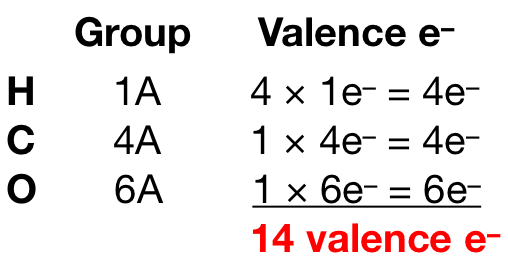# Problem: Sketch the following molecule: CH3OH (skeletal structure H3COH).

###### FREE Expert Solution

Hydrogen can only form 1 bond so it cannot be the central atom. Carbon (EN = 2.55) is less electronegative than oxygen (EN = 3.44) so carbon is the central atom. We then need to count the total number of valence electrons for each element:94% (131 ratings)###### Problem Details

Sketch the following molecule: CH3OH (skeletal structure H3COH).